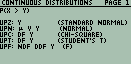## Upper Tail Probabilities

Description:Implements the calculation of upper tail probabilities for a number of distributions, including the normal, chi-squared, Snedecor's F, Student's t, the Binomial, the Negative Binomial, and the Poisson distribution. Extends the usable degrees of freedom for the F and t distribution by using large-sample normal approximations. Calculation of upper-tail probabilities of the Binomial, Negative Binomial, and the Poisson distribution use relationships between them and the F or t distribution.
Filename:uppertail422.zip
ID:6229
Current version:1.0
Author:Scott Hyde: http://jekyll.math.byuh.edu/
Size on calculator:4 KB
Platforms:48
User rating:Not yet rated (you must be logged in to vote)
Primary category:Math/Statistic
Languages:ENG
File date:2005/05/01 19:44:07
Source code:Included
Screenshot: